# Project Categories List

## Zen Variations 9

### Introduction

In ZV8 we dipped our toes into the waters of power JFET transistors using the new Lovoltech LU1014D in a simple circuit. The focus of the project was on the JFET itself, and except for a cascode transistor the rest of the amplifier used only passive components. Here in four installments we will increase the complexity of the circuitry around the JFET with an eye toward distortion performance surpassing any of the Zen projects to date.

Much of this project will make reference to ZV8 (AudioXpress, January 2006 and www.passdiy.com), which discusses the characteristics of the LU1014D power JFET and the circuit that forms the basis for this project. ZV8 also brought up the subject of load-line optimization, which we will exploit here, working the Drain to Source voltage (Vds) curve to reduce distortion.

For your quick reference, the most basic circuit is presented here as Figure 1.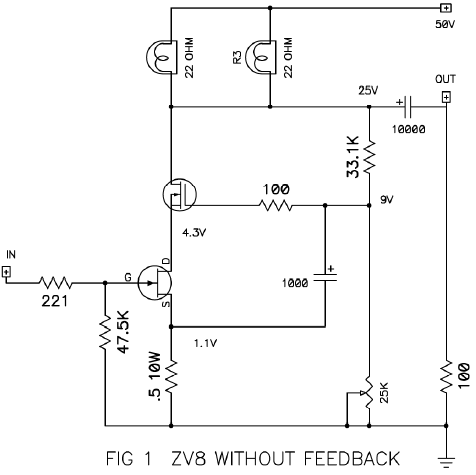Installment 1 – Supply Filter and Constant Current Source

We bias ZV8 with a pair of light bulbs, whose resistance offers about 11 ohms and delivers about 2.2 amps to the gain transistor. This has three drawbacks:

• 1) It is inefficient - the power supply must have about 50% higher voltage than the amplifier’s peak output.
• 2) The light bulb resistances parallel the load, so that an 8 ohm load looks like 4.6 ohms, making the gain device work nearly twice as hard and raising the distortion.
• 3) It has poor power supply noise rejection – a portion of the noise in the power supply comes through the light bulbs to the output.

First, we will put in an active supply filter to reduce power supply noise and turnon thump. Then we will replace the light bulbs with a 2 amp constant current source. It will not glow warmly on cold winter evenings, but it will draw less current, deliver about 50% more power, and has lower distortion and noise.

Figure 2 shows the replacement of the two light bulbs with the supply filter and the constant current source. Q4 is set up as a capacitance multiplier whose C3 and R8 time constant is set to 5 seconds. At this charge rate, the circuit will see minimal supply ripple and have a low turn-on transient. The supply voltage appearing on the Drain of Q3 enjoys some additional passive filtering from C2 and C6. Q4 drops about 4 volts off the supply, so that a 50V unregulated rail delivers about 46 volts at the Drain of Q3 (Voltages in all schematics are approximate). We could DC stabilize the supply with zener diodes across C3, but that would make it more difficult to have such a slow turn-on, so we will use the constant current source to DC stabilize the bias current.

The constant current source is formed by N channel Mosfet Q3 controlled by NPN transistor Q5. We make use of the physical constant of the PN junction of the Base-Emitter voltage of Q5 for a reference, and the circuit works to maintain about .66 volts across the resistors R4 through R6, which makes for a constant current of 2 amps. R10 through R13 are there to provide a relatively constant bias current for the Collector of Q5, with C4 bootstrapping from the output.

There are few other changes to the circuit of Figure 1, but you will note the addition of an audiophile-approved bypass capacitor on the output, and variability in the value of R3. Later we will see that that this value can be used to optimize the performance around the characteristics of individual JFETs.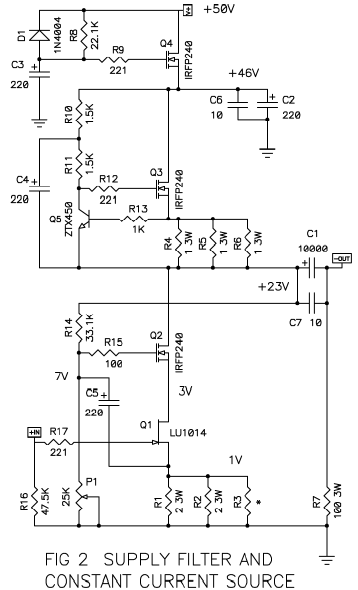In Figure 2 we trim the value of P1 for a Drain voltage of one-half the supply voltage by adjusting the Vds of the JFET – if the voltage on the Drain of Q3 is 46 volts, then we want about 23 volts on the Drain of Q2. With the appropriate value for R3 (approximately 2 ohms) we will see minimum distortion and a Vds across the JFET Q1 of 2 to 3 volts. This comes out to about 4 to 6 watts dissipation for the device.

This particular circuit is a transconductance amplifier, that is to say a current source whose output impedance is mostly determined by the 100 ohm output resistor R7. To lower the output impedance we will later apply some negative feedback.

Figure 3 shows the comparative performance of the circuits of Figure 1 and 2, and we see an immediate distortion reduction by about half. What is less clear is that the light bulb version is clipping at about 10% distortion at 10 watts, while the current source circuit clips comparably at about 20 watts.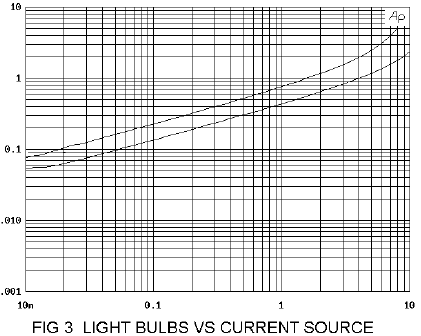### Installment 2 – Cascode Modulation

In ZV8 we spent some time discussing the triode-like character of the power JFET at low voltages, where both the transconductance and “plate” (Vds) characteristics show exponential behaviour. With such a device, you can exploit distortion cancellation by carefully choosing the load line of operation so that the variation in gain versus current is cancelled against the gain versus voltage so that the distortion of the device is dramatically reduced.

This phenomenon was only discussed in ZV8, and here is where we will put it to use. In many examples, correctly varying the Vds versus Ids (Drain-Source current) in the JFET would involve more complex circuitry, but on this rare occasion Mother Nature does us a favor. The optimal voltage variation can be had across resistors R1 – R3 which are coincidentally close to the value selected to give the JFET the correct DC self-biased value at 2 amps.

In Figure 2 C5 is placed so that the AC voltage at the Source of Q2 tracks the Source of Q1, holding the Vds of Q1 at a relatively constant DC value of about 2 to 3 volts. This is classic cascode operation. But a truly constant Vds for Q1 is not the lowest distortion load line for the device. Because we want to cancel two nonlinear characteristics, the optimal Vds across Q1 will be DC plus a finite AC voltage at around -600 mV per amp, which was determined by testing at 1 watt. In figure 4 we achieve this by grounding one leg of C5 - the simplest change imaginable. Figure 5 shows the difference.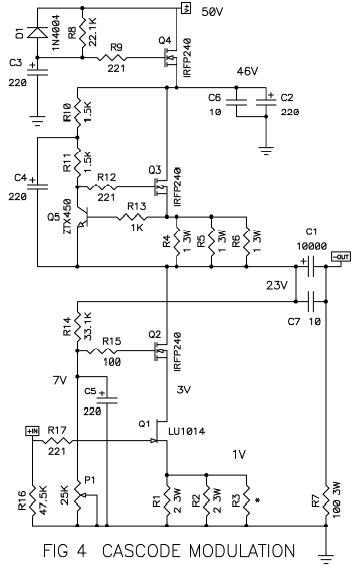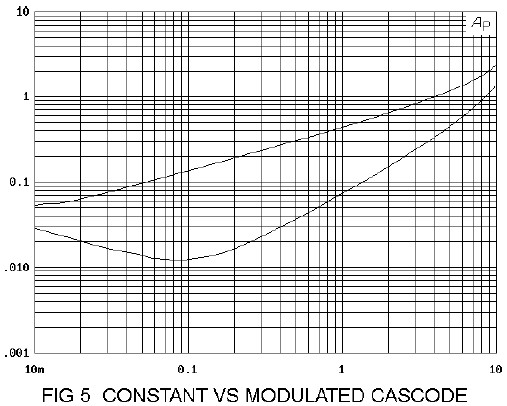### Installment 3 – Negative Feedback

The first two alterations improve the 1-watt performance by an order of magnitude, but it’s still a current source. You might elect to stop here if a current source meets your needs. If you want a damping factor, we will apply some loop feedback, as seen in Figure 6.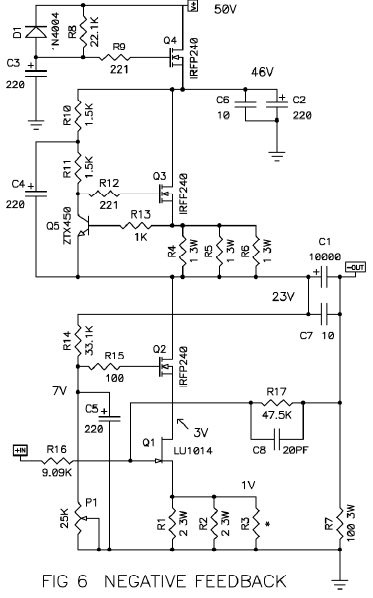Figure 6 is different from Figure 4 with R16, R17 and C8 forming a negative feedback loop. Like most of its Zen predecessors, ZV9 uses feedback to lower the output impedance. Roughly, R17 divided by R16 determines the gain and R16 determines the input impedance. With the values shown we use about 10 dB of feedback to get a damping factor of about 3 and a distortion reduction of about half. C8 imposes a small amount of compensation that makes the square wave look good. Figure 7 shows the comparison with and without feedback. Note that the improvement is not uniform – with simple low feedback circuits it often is not.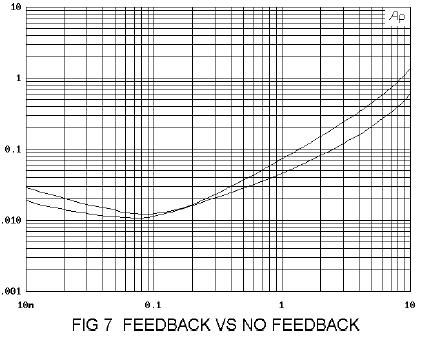### Installment 4 – The Aleph Current Source

Those familiar with ZV4 will probably figure out why I chose the particular current source of Figure 2 - it easily converts to the non-constant current source used in the Aleph series of amplifiers and as described in patent # 5,710,522.

ZV4 describes this current source in greater detail, but referring to Figure 8, the current source has the addition of C9, R18 and R19 that set the current source AC value to track an arbitrary fraction of the output. Usually we set this value around 50%, and we can use it to “ghost” the complex load impedance at a negative multiple of the load value. With the values chosen in Figure 8, an 8 ohm load would look like 16 ohms to the JFET gain device. By raising the apparent load impedance, the current source lowers the distortion of Q1 while still leaving it in control of the output. With tubes, variations of this idea have been called SRPP and SEPP output stages, although the distinction between an input and output stage is somewhat blurred in a single stage amplifier.

At one watt, the improvement is a factor of about 5, and at 10 watts a factor of about 10. Because the current source increases the open loop gain by about 6 dB, the damping factor jumps up to about 6, and the output gain goes up about a decibel or so. As the open loop gain is still finite, the input impedance is higher than the input resistor R16 at about 10 Kohm. The –3 dB bandwidth of the circuit is about 1 Hz to 80 KHz.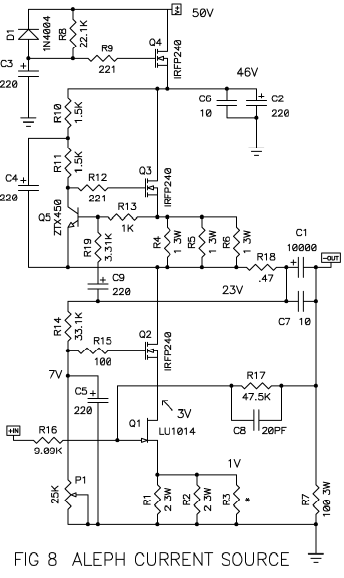Figure 9 shows the performance difference with and without the two resistors and capacitor that enable the Aleph current source at about 50% gain.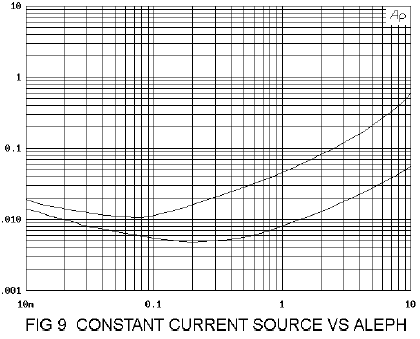### A Few Construction Notes

I made reference to choosing the value of R3 to get the lowest distortion. The best way to do this is to use a 5 ohm power potentiometer and measure the distortion for lowest possible value while adjusting P1 for output DC. This requires a distortion analyzer. Tweaking the various resistor values in the amplifier, I have seen 1-watt distortion as low as .003% at 1 watt.

Since most of you don’t have a distortion analyzer, I have an alternate method of determining the value of R3 based on the Vgs value of the Lovoltech LU1014 JFET in the test setup of figure 10. Using a 3 volt supply capable of greater than an amp, measure the Vgs of the device while the transistor case is still near room temperature. Most of them will sit between 1.0 and 1.1 volts, and you can use the graph to estimate the best value of R3 usually between 1 and 3 ohms.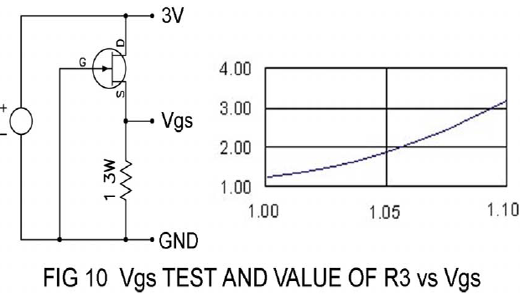As yet another alternative if you don’t want to test the devices, I recommend that you simply select R3 so that the Vds of the JFET in the amplifier is 2.5 volts with the output DC figure of 23 volts. More often than not, this is close to the sweet spot.

This amplifier idles at about 100 watts per channel, which means that each channel needs a heat sink rated at .25 deg C. per watt. The Lovoltech LU1014D requires significant heat sinking also, as will operate at 4 to 6 watts continuously. Because of the high dissipation on Q2 and Q3, I recommend mica insulators with thermal grease.

The characteristics of all the semiconductor devices in the circuit will drift a bit with temperature, so you will find yourself readjusting values as the circuit warms up to a steady state. This also indicates a short warm up time for the amplifier, which is generally less than an hour.

Unless otherwise specified, the schematics use ¼ watt metal film resistors and 50 volt capacitors, but you will note the 3 watt power resistors (I used the Panasonics from Digikey).

The Lovoltech devices are not yet in common distribution, and currently the best source for small quantities is the group buys arranged on www.diyaudio.com.

### Conclusion

When the first Zen amplifier was published 12 years ago, I did not particularly imagine the course the exploration would take. A single-stage amplifier is attractive on a number of levels - it serves as an excellent base for tutorial projects, partly because it isolates concepts involved in amplification but mostly because the simplicity invites beginners to try it.

Of course a single gain stage amplifier has a purely esthetic value. The challenge is to make the best simple amplifier possible and hope that the minimalist character of the signal chain allows the most information through with the least coloration.

Remarkably, we seem to have made real progress. The original Zen amplifier we measured .6% harmonic distortion at 1 watt, and here we see about 1/100th of that figure. I believe that the best correlation between measured performance and subjective listening occurs with simple circuits, giving rise to the obvious question - does this sound 100 times better?

No, only about 10 times better.